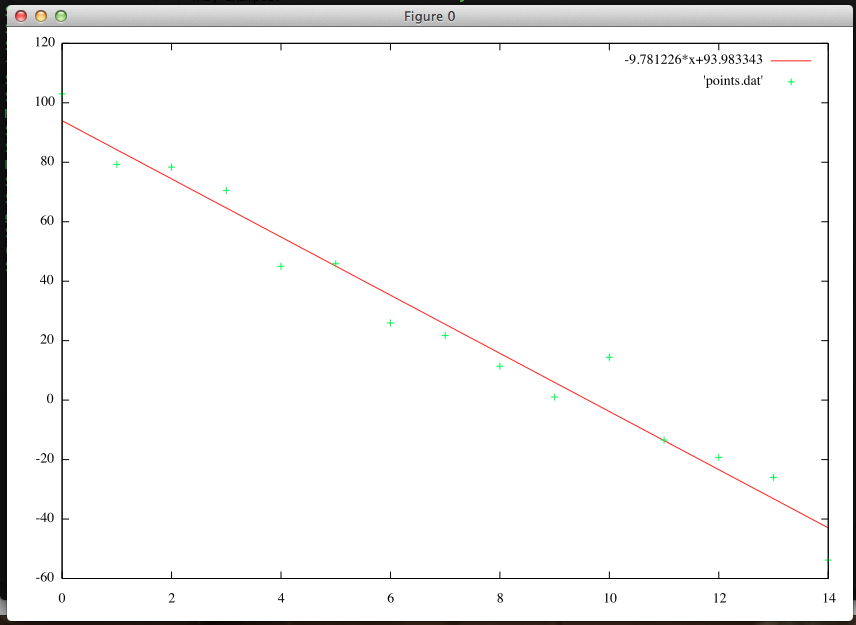# Linear Regression with Gradient Descent

I'm taking the Stanford Machine Learning class. The first algorithm we covered is Linear Regression using Gradient Descent. I implemented this algorithm is Python. Here's what it looks like:

import subprocess

def create_hypothesis(slope, y0):
return lambda x: slope*x + y0

def linear_regression(data_points, learning_rate=0.01, variance=0.001):
""" Takes a set of data points in the form: [(1,1), (2,2), ...] and outputs (slope, y0). """

slope_guess = 1.
y0_guess = 1.

last_slope = 1000.
last_y0 = 1000.
num_points = len(data_points)

while (abs(slope_guess-last_slope) > variance or abs(y0_guess - last_y0) > variance):
last_slope = slope_guess
last_y0 = y0_guess

hypothesis = create_hypothesis(slope_guess, y0_guess)

y0_guess = y0_guess - learning_rate * (1./num_points) * sum([hypothesis(point) - point for point in data_points])
slope_guess = slope_guess - learning_rate * (1./num_points) * sum([ (hypothesis(point) - point) * point for point in data_points])

return (slope_guess, y0_guess)

def plot_data_and_line(line, data_file):
# Plot with gnuplot
plot = subprocess.Popen(['gnuplot'], stdin=subprocess.PIPE)
line_eq = "%f*x+%f" % line
plot.communicate("plot %s, '%s' with points;" % (line_eq,data_file))

if __name__ == "__main__":
data_file = "points.dat"
points_str = [line.strip().split('\t') for line in open(data_file,'r').readlines()]
points = [(float(x),float(y)) for (x,y) in points_str]
line = linear_regression(points)
print line

plot_data_and_line(line, data_file)


This code takes in a data file with a data point on each line with the x and y separated by a TAB; Example:

0.000000    95.364693
1.000000    97.217205
2.000000    75.195834
3.000000    60.105519


This code also plots the data points and the resulting best-fit line in gnuplot. For example:This code, along with helper files, is on my github repo.